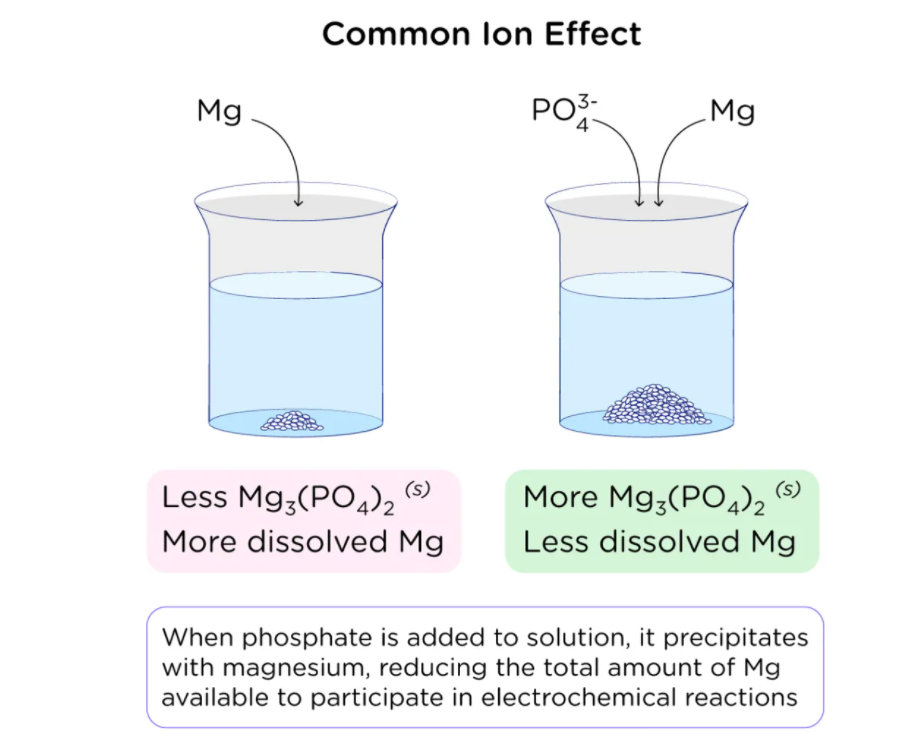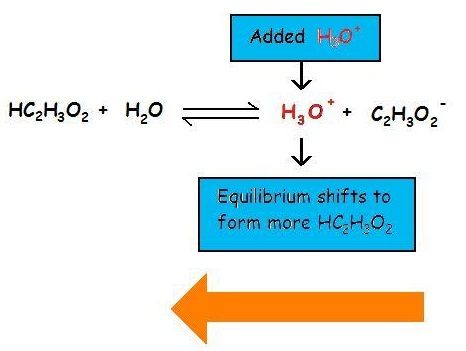# Common Ion Effect Study Guide

INTRODUCTION

One of the most important topics in chemistry is the concept of the common ion effect. In simpler terms, it is an effect that prevents one electrolyte from ionizing when another electrolyte is introduced. It is thought to be a result of Le Chatelier’s principle. The term “common-ion effect” refers to the influence on an equilibrium caused by adding an ion already present in the solution.### COMMON ION EFFECT IN CONTACT WITH WEAK ACIDS AND BASES

The addition of a common ion stops the weak base or weak acid from ionizing to an extent it would otherwise. The common ion effect prevents the ionization of a given weak acid by increasing the concentration of an ion which is a result of this equilibrium.### COMMON ION EFFECT ON SOLUBILITY

To know about the common ion effect on the extent of solubility is interesting. When a common ion is added, solubility drops as the reaction moves to the left to relieve the tension of the excess product. When a common ion is included in a dissociation process, the equilibrium shifts left, toward the reactants, resulting in precipitation.

### p-H and the Common Ion Effect

• Due to the common ion effect, when the conjugate ion of a buffer solution—a mixture of an acid and its conjugate base or an acid and its conjugate acid—is added to it, the pH of the buffer solution changes.
• Acetate ions are created when sodium acetate and acetic acid are both dissolved in the same solution.
• On the other hand, acetic acid only partially ionises while sodium acetate completely dissociates. This is true because sodium acetate is a strong electrolyte and acetic acid is a weak acid.
• Le Chatelier’s principle states that the additional acetate ions in sodium acetate help to suppress the ionisation of acetic acid, shifting the equilibrium to the left.
• The pH of the fluid rises as acetic acid dissociation is reduced.
• The shift in equilibrium brought on by the addition of an ion already participating in the equilibrium reaction is known as the Common Ion Effect.

AgCl(s) <=> Ag+(aq) + Cl-(aq)NaCl addition causes a left shift in this equilibrium.A mild acid/base system with the addition of a common ion:When A- (as a salt) is added to the reaction HA <=> H+ + A-The reaction is forced to the left and [H+] is decreased.

Example:

Add NaCH3COO, where acetate is the common ion, to the reaction.CH3COOH => H+ + CH3COO-

This drives the reaction to the left and lowers [H+] while increasing the concentration of the acetate ion. Since both processes decrease [H+], adding the common ion CH3COO- to a solution of CH3COOH is comparable to titrating the acid with NaOH. However, the common ion joins with protons to create more weak acid, rather than converting protons to water.

### Example of Common Ion Effect

When electrolytes are weak, the Common Ion effect is typically used to reduce the concentration of particular ions in the solution.

In the case of hydrogen sulphide, a weak electrolyte, this chemical experiences partial ionisation in an aqueous medium. This indicates that the ionised and component ions of the electrolyte are in an equilibrium state at a certain point:

H2S = H++ HS-

By using the law of mass action, it is possible to derive the equilibrium constant Ka’s value:

Ka = (H+) (HS-) / H2S

Along with potent acids like HCl, it starts to dissociate intoAnd H+ions: Cl-HCl H+ + Cl-

As the shared ion between the two chemicals, it causes an increase in the concentration of H+ ions. The equilibrium of H2S molecule ionisation then shifts to the left while Ka remains constant. Additionally, it causes a significant decrease in H2S dissociation. As a result, there are less sulphide ions in the solution due to the concentration of unionised H2S molecules.

### FAQs

1. What is meant by the common ion effect?

The common ion effect is the decrease in the extent of the solubility of an ionic precipitate caused by adding a soluble chemical having a common ion with the precipitate to the solution. Ideally, this behavior results from Le Chatelier’s principle for the equilibrium response of ionic association/dissociation.

2. What is an example of a common ion effect?

When sodium chloride (NaCl) is mixed in a solution of HCl & water, an instance of the common ion effect occurs. The products of the equilibrium between water and hydrochloric acid are H₃O and Cl-.

3. Where is the common ion effect used?

The common ion effect’s influence in solutions is most observable in the lowering of solid solubility. The solubility of a molecule often decreases with the addition of common ions due to a shift in equilibrium. The common ion effect is also prominent in buffer management. Buffering solutions comprise an acid or basic, as well as its conjugate equivalent. The addition of additional similar conjugate ions will eventually cause the pH of the solution to change. Even when evaluating solution equilibrium after including the ions already present in the solution, the common ion effect must be considered.

4. What are a few applications of the common ion effect?

The common ion effect is employed not only for quantitative chemical analysis but also for compound purification. If the concentration of any of the ions in a saturated solution is reduced, more salt dissolves, and the equilibrium moves to the right, according to Le Chatelier’s principle, until Ksp equals Qsp.

When the concentration of any of the ions is raised by adding an electrolyte, the equilibrium moves to the left according to Le Chatelier’s principle until Ksp = Qsp. For gravimetric measurement, this effect is utilized to entirely precipitate one of the ions in the form of its sparingly soluble salt having a low solubility product.

We hope you enjoyed studying this lesson and learned something cool about Common Ion Effect! Join our Discord community to get any questions you may have answered and to engage with other students just like you! Don’t forget to download our App to experience our fun VR classrooms – we promise, it makes studying much more fun! 😎

]]>